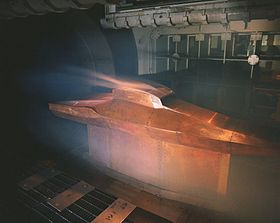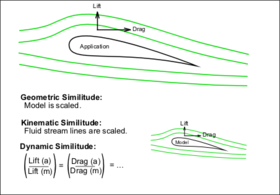# Similitude (model)

Similitude (model)A full scale X-43 Wind tunnel test. The test is designed to have dynamic similitude with the real application to ensure valid results.

Similitude is a concept applicable to the testing of engineering models. A model is said to have similitude with the real application if the two share geometric similarity, kinematic similarity and dynamic similarity. Similarity and similitude are interchangeable in this context.

The term dynamic similitude is often used as a catch-all because it implies that geometric and kinematic similitude have already been met.

Similitude's main application is in hydraulic and aerospace engineering to test fluid flow conditions with scaled models. It is also the primary theory behind many textbook formulas in fluid mechanics.

## Overview

Engineering models are used to study complex fluid dynamics problems where calculations and computer simulations aren't reliable. Models are usually smaller than the final design, but not always. Scale models allow testing of a design prior to building, and in many cases are a critical step in the development process.

Construction of a scale model, however, must be accompanied by an analysis to determine what conditions it is tested under. While the geometry may be simply scaled, other parameters, such as pressure, temperature or the velocity and type of fluid may need to be altered. Similitude is achieved when testing conditions are created such that the test results are applicable to the real design.The three conditions required for a model to have similitude with an application.

The following criteria are required to achieve similitude;

• Geometric similarity – The model is the same shape as the application, usually scaled.
• Kinematic similarity – Fluid flow of both the model and real application must undergo similar time rates of change motions. (fluid streamlines are similar)
• Dynamic similarity – Ratios of all forces acting on corresponding fluid particles and boundary surfaces in the two systems are constant.

To satisfy the above conditions the application is analyzed;

1. All parameters required to describe the system are identified using principles from continuum mechanics.
2. Dimensional analysis is used to express the system with as few independent variables and as many dimensionless parameters as possible.
3. The values of the dimensionless parameters are held to be the same for both the scale model and application. This can be done because they are dimensionless and will ensure dynamic similitude between the model and the application. The resulting equations are used to derive scaling laws which dictate model testing conditions.

It is often impossible to achieve strict similitude during a model test. The greater the departure from the application's operating conditions, the more difficult achieving similitude is. In these cases some aspects of similitude may be neglected, focusing on only the most important parameters.

The design of marine vessels remains more of an art than a science in large part because dynamic similitude is especially difficult to attain for a vessel that is partially submerged: a ship is affected by wind forces in the air above it, by hydrodynamic forces within the water under it, and especially by wave motions at the interface between the water and the air. The scaling requirements for each of these phenomena differ, so models cannot replicate what happens to a full sized vessel nearly so well as can be done for an aircraft or submarine—each of which operates entirely within one medium.

Similitude is a term used widely in fracture mechanics relating to the strain life approach. Under given loading conditions the fatigue damage in an un-notched specimen is comparable to that of a notched specimen. Similitude suggests that the component fatigue life of the two objects will also be similar.

## An example

Consider a submarine modeled at 1/40th scale. The application operates in sea water at 0.5 °C, moving at 5 m/s. The model will be tested in fresh water at 20 °C. Find the power required for the submarine to operate at the stated speed.

A free body diagram is constructed and the relevant relationships of force and velocity are formulated using techniques from continuum mechanics. The variables which describe the system are:

Variable Application Scaled mode Units
L (diameter of submarine) 1 1/40 (m)
V (speed) 5 calculate (m/s)
ρ (density) 1028 998 (kg/m3)
μ (dynamic viscosity) 1.88x10−3 1.00x10−3 Pa·s (N s/m2)
F (force) calculate to be measured N   (kg m/s2)

This example has five independent variables and three fundamental units. The fundamental units are: metre, kilogram, second.

Invoking the Buckingham π theorem shows that the system can be described with two dimensionless numbers and one independent variable.

Dimensional analysis is used to re-arrange the units to form the Reynolds number (Re) and pressure coefficient (Cp). These dimensionless numbers account for all the variables listed above except F, which will be the test measurement. Since the dimensionless parameters will stay constant for both the test and the real application, they will be used to formulate scaling laws for the test.

Scaling laws:$R_e = \left(\frac{\rho V L}{\mu}\right)$$\longrightarrow$$V_{model} = V_{application} \times \left(\frac{\rho_a}{\rho_m}\right)\times \left(\frac{L_a}{L_m}\right) \times \left(\frac{\mu_m}{\mu_a}\right)$$C_p = \left(\frac{2 \Delta P}{\rho V^2}\right), F=\Delta P L^2$$\longrightarrow$$F_{application} =F_{model} \times \left(\frac{\rho_a}{\rho_m}\right) \times \left(\frac{V_a}{V_m}\right)^2 \times \left(\frac{L_a}{L_m}\right)^2$

This gives a required test velocity of:$V_{model} = V_{application} \times 21.9$ .

The force measured from the model at that velocity is then scaled to find the force that can be expected for the real application:$F_{application} = F_{model} \times 3.44$

The power P in watts required by the submarine is then:$P [\mathrm{W}] =F_{application}\times V_{application}= F_{model} [\mathrm{N}] \times 17.2 \ \mathrm{m/s}$

Note that even though the model is scaled smaller, the water velocity needs to be increased for testing. This remarkable result shows how similitude in nature is often counterintuitive.

## Typical applications

Similitude has been well documented for a large number of engineering problems and is the basis of many textbook formulas and dimensionless quantities. These formulas and quantities are easy to use without having to repeat the laborious task of dimensional analysis and formula derivation. Simplification of the formulas (by neglecting some aspects of similitude) is common, and needs to be reviewed by the engineer for each application.

Similitude can be used to predict the performance of a new design based on data from an existing, similar design. In this case, the model is the existing design. Another use of similitude and models is in validation of computer simulations with the ultimate goal of eliminating the need for physical models altogether.

Another application of similitude is to replace the operating fluid with a different test fluid. Wind tunnels, for example, have trouble with air liquefying in certain conditions so helium is sometimes used. Other applications may operate in dangerous or expensive fluids so the testing is carried out in a more convenient substitute.

Some common applications of similitude and associated dimensionless numbers;

 Incompressible flow (see example above)– Reynolds number, Pressure coefficient, (Froude number and Weber number for open channel hydraulics) Compressible flows – Reynolds number, Mach number, Prandtl number, Specific heat ratio Flow-excited vibration Strouhal number Centrifugal compressors – Reynolds number, Mach number, Pressure coefficient, Velocity ratio Boundary layer thickness – Reynolds number, Womersley number, Dynamic similarity

Wikimedia Foundation. 2010.

### Look at other dictionaries:

• Similitude — may refer to:* Similitude ( Enterprise episode), an episode of the television series Star Trek: Enterprise * Similitude (geometry), a concept in Euclidean geometry * Similitude (model), a concept used in the testing of engineering models …   Wikipedia

• Model — Contents 1 Physical 1.1 Human models 2 Nonphysical 2.1 …   Wikipedia

• Similitude of ship models — Manned models Many research workers, hydraulics specialists and engineers have used scale models for over a century, in particular in towing tanks. Manned models are small scale models that can carry and be handled by at least one person on an… …   Wikipedia

• Model (physical) — A physical model is a smaller or larger physical copy of an object. The object being modelled may be small (for example, an atom) or large (for example, the Solar System).The geometry of the model and the object it represents are often similar in …   Wikipedia

• model — Synonyms and related words: Geistesgeschichte, Hegelian idea, Kantian idea, Miss America, Platonic form, Platonic idea, admirable, aesthetic form, apotheosis, archetypal, archetype, archetypical, arrangement, art form, assemble, barometer,… …   Moby Thesaurus

• similitude — Synonyms and related words: accordance, affinity, agreement, alikeness, allegory, alliance, ally, alter ego, analogon, analogue, analogy, aping, approach, approximation, assimilation, associate, balancing, brother, certified copy, close copy,… …   Moby Thesaurus

• similitude — n 1. resemblance, likeness, similarity; agreement, concordance, equivalence, coincidence, comparability, correspondence, congruity, conforma bility. 2. counterpart, match, image, semblance; representation, depiction, portrayal, delineation;… …   A Note on the Style of the synonym finder

• Physical model — Model of a burning house at Bekonscot, Beaconsfield, UK …   Wikipedia

• Boeing Model 6D — Model 6D Constructeur Boeing Type Hydravion Premier vol avril 1928 …   Wikipédia en Français

• Ship model — This article is concerned primarily with static models. For operating models see model yachting or radio controlled boat, Model of a 19th century vessel in the Bishop Museum, Hawaii …   Wikipedia